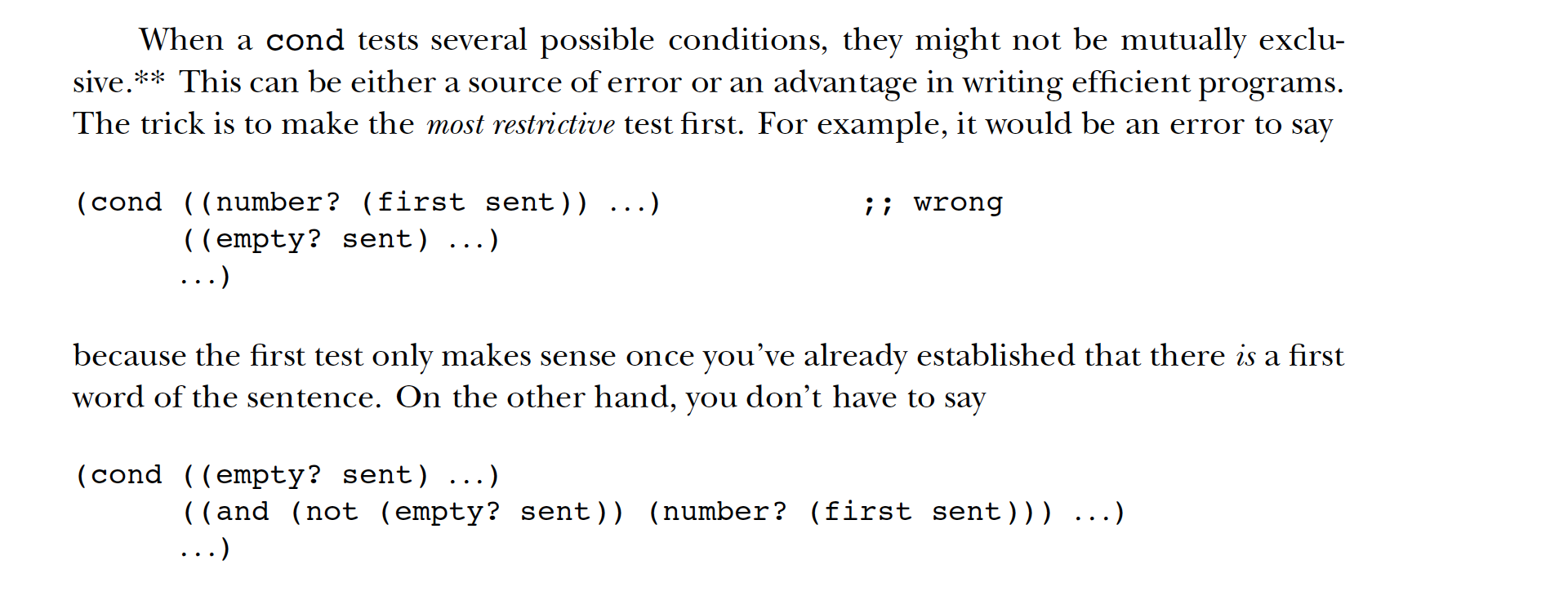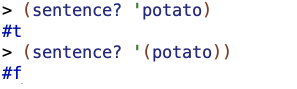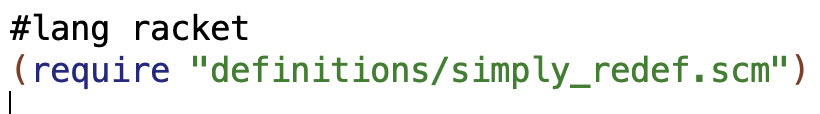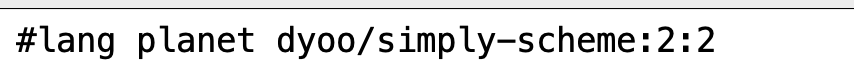# Simply Scheme Chapter 6 – True and False

Quotes from here or elsewhere in Simply Scheme unless otherwise specified. Refers to “the book” or “SS’ are to Simply Scheme.

Sometimes I show past solutions from previous attempts through the book. Those are for comparison only – I’m not really testing those like I am my current solutions.

### Predicates

Boolean values in scheme are `#t` for true and `#f` for false (and `#true` and `#false` also seem to work).

A function that returns either true or false is a predicate. Some examples:
`equal?`– checks if args are equal (works on numbers, sentences, procedures, words, booleans).
`member?` – checks if a letter is in a word or a word is in a sentence.
`=` – checks if numbers are equal.
`>` – checks if one number is greater than another. Similar functions are `<`, `>=`, and `<=`.
`empty?` – checks if for an empty sentence `'()` or string `""`
`number?` – checks if an argument is a number.
`boolean?` – checks if an argument is a boolean.
`word?` – checks if an argument is a word.
`sentence?` – checks if an argument is a sentence.

You can also define your own predicates e.g.

The `and`, `or`, and `not` procedures, along with `if`, are useful to use in conjunction with predicates. The book gives some examples.

### Special Forms

`if`, `and`, and `or` are special forms.

`if` doesn’t evaluate all its arguments. It only evaluates the one it needs to based on whether the first argument returns `#t` or not.

The rule is that if always evaluates its first argument. If the value of that argument is true, then if evaluates its second argument and returns its value. If the value of the first argument is false, then if evaluates its third argument and returns that value.

So in the above example, `if` evaluates the first argument, which is `(= 3 3)`. If and only if that returns true does it evaluate and return the second argument `'sure`. If and only if the first argument returns false does it evaluate `(factorial 1000)`.

`and` and `or` are also special forms which evaluate their arguments from left to right. `or` stops and returns a true value as soon as it hits a true argument, and `and` stops and returns false as soon as it hits a false argument.

### Semipredicates

SS says every value is considered true except `#f`. This fact allows us to have semipredicates. The book doesn’t actually formally define what a semipredicate is in this chapter. But I think that predicates are procedures that return either true or false, and semipredicates seem to be procedures that can return either false or a true value other than just`#t` or `#true`.

#### 🤔 Integer-quotient and Some Confusion

There was a passage that I had some trouble understanding. This might be a bit rambly so maybe skip it if you’re just quickly skimming.

`#T` isn’t the only true value. In fact, every value is considered true except for `#f`.

This allows us to have semipredicates that give slightly more information than just true or false. For example, we can write an integer quotient procedure. That is to say, our procedure will divide its first argument by the second, but only if the first is evenly divisible by the second. If not, our procedure will return `#f`.

SS then gives the following example (i’ve added the `divisible` procedure below, which was defined earlier in the chapter, as necessary context)

So backing up, I thought that, in the part that says…

This allows us to have semipredicates that give slightly more information than just true or false.

…that the “this” referred to the fact that…

every value is considered true except for `#f`

…which I will refer to herein as the Truth-Fact. Based on that interpretation, I expected the Truth-Fact to be a necessary part of how the first version of `integer-quotient` works. I now think that maybe they were actually referring to the Truth-Fact being necessary for the revised version that they describe later in the section, though, but I’m not sure.

I read the procedure above this way: `divisible` is a necessary helper procedure. It relies on the `=` procedure, which returns `#t` if the remainder is `0` and `#f` if the remainder is something other than 0. The `divisible?` procedure called within the first argument of the `if` will return `#t` if the remainder of the first argument passed into `integer-quotient` is 0 when divided by the second argument. In that case, the check by the `if` procedure that its first argument is true will succeed, and the second argument will be evaluated and the quotient returned. If `divisible?` returns false, however, then the third argument to the `if` – the `#f` – will be returned.

With that description of the procedure in mind, I don’t see how the Truth-Fact plays a role in the version of `integer-quotient` above. I get that integer-quotient meets what I laid out as my definition of a semipredicate, but whether something is a semipredicate seems different than whether it relies on the Truth-Fact for its operation.

`and` and `or` also semipredicates. The book mentions that `or` will return the first true result:

`3` has a truth value of `#t`. `or` doesn’t return that `#t`, but the value itself.

`and` works the following way:

If all the arguments given to `and` are true, it returns the value of the last argument.

Of the fact that `and` returns the value of the last argument when all the arguments given to `and` are true, the book says the following:

As an example in which this behavior is useful, we can rewrite integer-quotient more tersely:

With this example, I can easily see how the Truth-Fact is necessary to how it works. Specifically, the programmer is relying on the fact that the numeric value of `(/ big little)` will return as true (for the `and`) and return as the value that the procedure outputs. When the first argument to the `and` (the `(divisible? big little)`) is returned as true, the programmer wants the second argument to be the value that is returned by invocation the procedure. `and` will return the final value when both arguments are true, and so the number returned by `(/ big little)` being true is necessary for this function to work in the way the programmer wants it to.

### Cond

`cond` provides a concise way of handling situations where you have to choose from among multiple possibilities.

To take one example, for `((equal? letter 'i) 1)`, `(equal? letter 'i)` is the condition that gets tested, and `1` is the thing that gets returned if the condition is true. So each `cond` clause like this is analogous to the first two arguments after an `if` clause. But with `cond` you get to have a whole series of alternatives rather than just specifying one like you can in `if`, and each alternative can have its own condition, unlike in `if` where you’re just testing the first argument.

`cond` is a special form and doesn’t evaluate arguments until it needs to.

Re: `else`:

By convention, the last argument always starts with the word `else` instead of an expression. You can think of this as representing a true value, but `else` doesn’t mean true in any other context; you’re only allowed to use it as the condition of the last clause of a `cond`.

The book emphasizes that one should put the most restrictive test first in a `cond` statement. Pasting this as images cuz of a formatting issue on the web page:Why is `(empty? sent)` more restrictive than `(number? (first sent))`? I think because `(empty? sent)` will only return true on an empty sentence, whereas `(number? (first sent))` will return true on infinitely many potential sentences. So `(empty? sent)` rules out all sentences but one, whereas `(number? (first sent))` will return true for many sentences. Another way of saying it is that `(empty? sent)` is trying to find out whether we’re dealing with a sentence with content at all, whereas `(number? (first sent))`is trying to get some information about what sort of non-empty sentence we’re dealing in within the universe of non-empty sentences.

The book emphasizes that the values returned by `if` can be used as part of larger functions, consistent with Scheme’s overall approach. E.g. this works:

### Boring Exercises

#### Exercise 6.1

##### ✅ Exercise 6.1.1

What values are printed when you type these expressions to Scheme? (Figure it out in your head before you try it on the computer.)

This returns `'(nowhere man)`, as I expected.

##### ❌ Exercise 6.1.2

I misread how `cond` and this program work. I expected it to return 49 for some reason – I think that I thought the first line would have `(empty?)` evaluate `3` and return `#f`, and so then `(square 7)` would evaluate and return the true value `49`. What actually happened was that the program returned `3`.

The book actually has a relevant example. Let me reread that:

Don’t get into bad habits of thinking about the appearance of `cond` clauses in terms of “two parentheses in a row.” That’s often the case, but not always. For example, here is a procedure that translates Scheme true or false values (`#t` and `#f`) into more human-readable words `true` and `false`.

So what is happening? In this example, when the `value` is something that evaluates to `#t`, the `'true` is returned. The `value`, despite not being a complex expression like `((equal? letter 'i) 1)`, has a truth value, and so when `cond` sees that that value is `true`, it then proceeds to return the “consequent”, which in this case is the word `'true`.
In all other cases, `truefalse` returns the word`false`.

I think the point is that`cond` can, but need not have, a sub-expression as the first value which gets checked for its truth value. It can be a simple expression like `value` above. Or a procedure like `empty?`! Since basically everything in Scheme is true, `empty?` has a truth value too..So in the `cond` for this exercise, `empty?` gets evaluated as true, and so the consequent `3` gets returned as a result.

##### ✅ Exercise 6.1.3

This returns `goes`.

#### ✅ Exercise 6.2

What values are printed when you type these expressions to Scheme? (Figure it out in your head before you try it on the computer.)

##### ✅ Exercise 6.2.1

This returns `#t`.

##### ✅ Exercise 6.2.2

This returns `#f`.

##### ✅ Exercise 6.2.4

This returns `#t`.

##### ✅ Exercise 6.2.5

This returns `#t`.

##### ✅ Exercise 6.2.6

this returns `#t`.

#### ✅ Exercise 6.3

Rewrite the following procedure using a `cond` instead of the `if`s:

#### ✅ Exercise 6.4

** Rewrite the following procedure using an `if` instead of the `cond`:

### Real Exercises

#### ✅ Exercise 6.5

Write a procedure `european-time` to convert a time from American AM/PM notation into European 24-hour notation. Also write `american-time`, which does the opposite:NOTE: for `((equal? (first (bf us-time)) 'am) (first us-time))`, replacing `(first (bf` with `last`, like AnneB does in her implementation, would be better…

##### Past Solution

Old answer, from a previous time I did the book:

For `european-time`, I think checking for whether the entire input is `equal?` to the `us-time` for the special cases of 12am and 12pm makes way more sense and is much more elegant and readable than the stuff i did last time with `member?` etc.

#### ✅ Exercise 6.6

Write a predicate `teen?` that returns true if its argument is between 13 and 19.

AnneB points out that the `if` statement is not necessary and this can be represented as:

##### Past Solution

Last time I went through Simply Scheme I did the following for this problem:

This works but would scale up really badly if you wanted to do a similar sort of thing but with a larger range of numbers.

#### ✅ Exercise 6.7 – Troubleshooting Scheme 🧐

Write a procedure `type-of` that takes anything as its argument and returns one of the words `word`, `sentence`, `number`, or `boolean`:

For this program I had to change the Scheme file I was using. I had to do this cuz the `sentence?` procedure was returning erroneous results.And I had been using this declaration at the beginning of my Scheme files:(I kept the `simply_redef.scm` file in a `definitions` subfolder in each place where I was storing .scm files).

For this exercise, I switched to using this declaration:This caused `sentence?` to perform as expected 🙂So with that out of the way, my actual answer to the exercise:

test inputs:

outputs:

#### ✅ Exercise 6.8

Write a procedure `indef-article` that works like this:

Don’t worry about silent initial consonants like the `h` in `hour`.

#### ✅ Exercise 6.9

Sometimes you must choose the singular or the plural of a word: `1 book` but `2 books`. Write a procedure `thismany` that takes two arguments, a number and a singular noun, and combines them appropriately:

In the court of doing this assignment, I greatly improved `plural`.

Answer. `plural` is where the interesting action is happening here. Note: some of the options in the upper-right of the code window may help with readability.

#### ✅ Exercise 6.10

Write a procedure `sort2` that takes as its argument a sentence containing two numbers. It should return a sentence containing the same two numbers, but in ascending order:

Again I weirdly don’t make use of `last`.

#### ❌ Exercise 6.11

NOTE: caught an error in this program after posting and changed the ✅ to an ❌. I discuss the error here

Write a predicate `valid-date?` that takes three numbers as arguments, representing a month, a day of the month, and a year. Your procedure should return `#t` if the numbers represent a valid date (e.g., it isn’t the 31st of September). February has 29 days if the year is divisible by 4, except that if the year is divisible by 100 it must also be divisible by 400.

I defined a program that looked for violations of the date rules and returned false if it found a violation, but returned true otherwise. I’ve included versions with both no comments and comments in case the commented version has readability issues. Note: some of the options in the upper-right of the code window may help with readability.

AnneB addressed some issues related to year-validity that I didn’t.

##### Past Solution 1

Here’s an earlier attempt of mine at solving this problem.

The organization seems solid. I like how concise my current solution is though.

#### ✅ Exercise 6.12

Make `plural` handle correctly words that end in `y` but have a vowel before the `y`, such as `boy`. Then teach it about words that end in `x` (box). What other special cases can you find?

I did this in an earlier problem.

I’ll do it clean here without the comments

AnneB‘s does more cases than mine does!

#### ✅ Exercise 6.13

Write a better `greet` procedure that understands as many different kinds of names as you can think of:

Answer. My `remove-post-nominal-letters` procedure handles a bunch of cases like the

Note: my previous work on this problem in past attempts didn’t deal with the MLK jr case at all.

I really like how buntine organized his solution here with the helper procedures.

#### ✅ Exercise 6.14

Write a procedure `describe-time` that takes a number of seconds as its argument and returns a more useful description of that amount of time:

I should use the composability if `if` more to make stuff more concise.